American Journal of Chemical Engineering
Volume 3, Issue 1, January 2015, Pages: 19-24

Simulation of the Solubility of SO2 on Water Using CFD Modeling Airlift Reactor

2Humanities Research Cluster, University of Malaya, Kuala Lumpur, Malaysia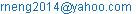(R. R. AL-Hussari),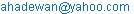(A. A. AL-Fatlawy),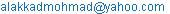(M. H. Al-Aqad)

Rana R. AL-Hussar, Ahad D. AL-Fatlawy, Mohammed H. Al-Aqad. Simulation of the Solubility of SO2 on Water Using CFD Modeling Airlift Reactor. American Journal of Chemical Engineering. Vol. 3, No. 1, 2015, pp. 19-24. doi: 10.11648/j.ajche.20150301.12

Abstract: Nowadays, petroleum and power plants emissions are increasingly recognized as a serious, worldwide public concern. In recent years, there has been an increasing interest to find solutions to decrease SO2 and the sulfur dioxide emissions from petroleum and electricity facilities. So far, several attempts have been made to figure out methods, procedures or techniques to lessen the emissions of SO2 which requires high cost to develop these techniques. However, the current research provides a solution to this issue through simulation, which allows new ways to test and diagnose these methods, procedures with low cost and danger. Nevertheless, solubility of SO2 in water may consider as conventional approach while sulfur dioxide of SO2 is a soluble gas in water, which can be oxidized through cloud and fog by producing sulfuric acid. Finally, this paper offers a new way to test SO2 solubility through using CFD modeling Airlift reactors.

Keywords: Airlift, CFD, Velocity, SO2, Bubble, Column, Holdup, Gas, Liquid

Contents

1. Introduction

Airlift loop reactors have emerged as one of the most promising devices in chemical, biochemical and environmental engineering operations. Its main advantages over conventional reactors include excellent contact among different phases, ease of removal or replenishment of particles, and high heat and mass transfer rates (Zhang, et. al, 2006). Airlift reactors are reaction vessels divided into two main sections ± the riser, where the gas is usually injected, and the down comer. As a result of the different gas holdup in the riser and in the downcomer, the bulk density of the fluid in these zones is different and liquid circulation is induced. (Freitas and Teixeira, 1998)

Gas holdup and liquid circulation velocity are amongst the most widely studied parameters in airlift reactors. Clearly, all aspects of performance of airlift systems are influenced by gas holdup and liquid circulation (Chistiet. al., 1998).

The numeric simulation in Fluid Mechanics ,Heat and Mass Transfer, commonly known as CFD "Computational Fluid Dynamics", has an expressive development in the last 20 years as a tool for physical problem analyses in scientific investigations, and nowadays as a powerful tool in solving important problems applied to engineering CFD permits a detailed investigation of local effects of different types of equipment, such as chemical and electrochemical reactors, heat exchangers, mixing tanks, cyclones, combustion systems, among others (Silva et. al.,2005). For the numerical computation of two-phase flows two approaches are mainly applied, namely the Euler/Euler and the Euler/Lagrange approach. The first method considers both phases as interacting continua, while in the second method the discrete nature of the dispersed phase is taken into account by tracking a large number of individual bubbles through the flow field (Lain et.al. 2000).

An increasing number of papers deal with CFD application to bubble columns. Where3D simulation of bubble column; gas holdup was calculated by plotting the distribution of gas volume fraction vs. column height(Bohn, 2000).Also commercial code CFX 4.3 used to simulate the gas-liquid flow in 3D rectangular bubble columns. The movement of a bubble plume in a 3D bubble column was also simulated where in contrary to the other case only little temporal behavior was found (Deenet. al. 2000). CFD simulations of the bubble column have been performed using several multiphase approaches, which performed to study the effects of superficial gas velocity, sparger configuration (including bubble diameter), and the height to width (H/W) ratio of the column on the low frequency oscillations and time averaged flow variables, such as vertical liquid velocity and gas holdup (Buwa and Ranade, 2003).

2. Theory

In the present work some assumptions were made in the development of the mathematical model: The airlift reactor (SO2 (gas)/water system) consists in four sections (riser, downcomer, top and the bottom sections; gas and liquid phase)with a constant density for the entire reactor; for the internal-loop reactor. The process operates sat constant pressure and temperature.

2.1. CFD Model

In present work Eulerian simulations were carried out for an airlift reactor SO2/water system using 3-D CFX-5, shown schematically in figure (1).Where the column height 77cm, and radius 8cm with sparger (radius 0,4cm).Above the base of column 3cm and the draft tube(height 70cm, 6.5cm) above the column base 3.5cm. The gas velocity, ug, at the bottom inlet was varied in range (1 to 7 with ramp 2 m/s).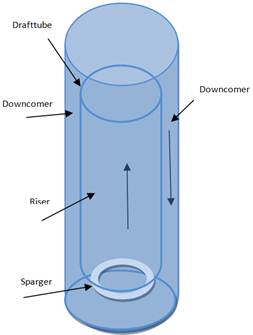Figure (1). Schematic of airlift reactor.

2.2. Mathematical Models

The governing equations describe the CFD calculations performed in this research. The multi-fluid model section describes the general formulation of the model equations. The multi fluid model will be used to setup Euler- Euler simulations.

In Euler-Euler simulations, separate phases are treated as interpenetrating fluids. This means that at a certain position, all phases can be present with a certain volume fraction, and no clear interface between the phases can be established. This allows both the length and the time scale on which these equations are being solved. The only body force taken into account in this research is gravitational force: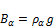(1)

2.2.1. Multi-Fluid Model

The general scalar advection diffusion equation: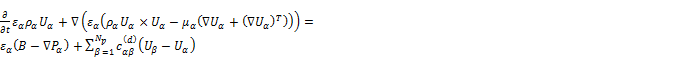(2)

The continuity equation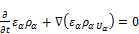(3)

And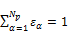(4)

The formulas above define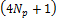equations for the following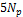unknowns: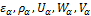For this system of equations to be solved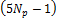more equations need to be added. In this research, the additional equation defines that all phases share the same pressure field (Van Baten, 2000):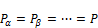(5)

2.2.2. Drag

Drag models defines how momentum is being transferred if a difference in velocity is present between two phases. In the multi fluid model, interphase momentum transfer can be modeled by specifying a value for the interphase momentum transfer coefficients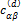equation (2) (Van Baten, 2000).

2.2.3. Particle Model

The particle model models the interphase momentum transfer between a continuous phase and a disperse phase (van Baten, 2000):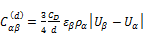(6)Or, alternatively (only if explicitly mentioned), a modification of equation (15) that takes into account the holdup of the continuous phase (Van Baten, 2000):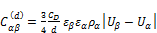(7)

In the above, the drag coefficient CDis a model parameter and d represents the average size of the particles or bubbles that make up phase.

In this research, the drag coefficient CD is based on the distorted flow regime (the intermediate regime between spherical bubbles and spherical cap bubbles)(Van Baten, 2000):(8)(9)

2.3. Flow Regime

Bubble column flow regimes are broadly classified as homogeneous and heterogeneous flows (Krishna and Baten, 2003).

Observed change from bubbly flow to transition flow is asymptotic depending on various factors [Wallis, 1969] which affect the size of the gas bubble by altering the degree of coalescence. The flow regime transition is normally identified based on instability theory, analysis of fluctuation signals, and the drift flux model. Higher gas density is found to have a stabilizing effect on the flow and that the gas fraction at the instability point (i.e., transition point) increases with gas density, while the gas velocity at the instability point only slightly increases with gas density. The drift flux of gas increases with the gas holdup in the dispersed regime; in the coalesced bubble regime, the rate of increase is much larger (Zhe Cui, 2005).

In this method, the drift flux, jGL (the volumetric flux of either phase relative to a surface moving at the volumetric average velocity) is plotted against the superficial gas velocity, uG. The drift flux velocity is given by: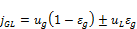(10)

Where εg is gas holdup and uLis superficial liquid velocity. The positive or negative sign indicates counter-current or co-current flow of liquid relative to the gas phase, respectively. In the case of stationary liquid phase (uL= 0) this equation simplifies to: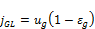(11)

The change in the slope of the curve of the drift flux versus gas holdup represents the transition from homogeneous to heterogeneous flow.

2.4. Interfacial Area Density

The interfacial area density (or concentration) is defined as the sum of the interfaces per unit volume of two-phase mixture. This term appears in basic conservation equations in two-fluid model formulations. In order to close the equation system, this term is usually given as a closure law. With an assumption of spherical bubbles, the local interfacial area concentration αi is given as (van Baten, 2000):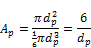For particle model a dispersed phase, p , with volume fraction,, the particle model estimates the interfacial area concentration,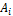as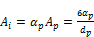(12)

3. Result and Discussion

Recent publications have shown that hydrodynamics of airlift reactors and bubble column can be estimated with computational fluid dynamic simulations based on Eulerian equations. We are interest to work at homogenize region because the specifications of bubble (size, number) small size, large no. in order to get large interfacial area for best mass transfer. So that for all flow processes in the reactor; setting a constant value of bubble size means that neglecting effects of coalescence, bubble-breakup due to hydrostatic pressure which decrease with increasing vertical position in the reactor and thus can be held responsible for the model’s actual inability to account for the flow regime transitions observed in the measurements.

The Following snap-shots in figure (2) show 3D axis-symmetric simulations results for gas holdup at different inlet gas velocities. The colors depict gas holdup according to the scale shown on the left. However,in draft tube the intermediate compound decrease the possibility of decomposes with high velocity because of low retention time. Also Volume fraction for SO2 approximately identical between two regions degassing zone (outlet) and draft tube region because high percentage of SO2 is dissolve in water at this region without decomposing (intermediate compound) during raising in column.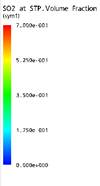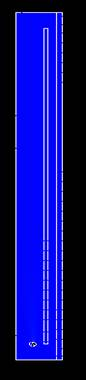Ug= 1m/s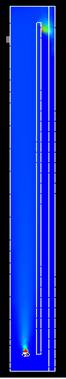Ug=3 m/sUg=5/s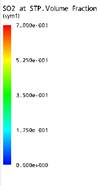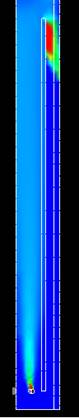ug=7m/s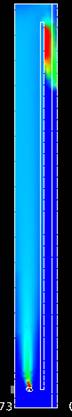ug=8m/s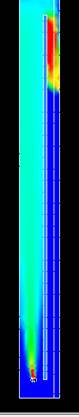ug=9m/s

Figure (2). Contours of air volume fraction.

The gas holdup in down comer remains lower than that in the riser as shown in figure (3), the difference in the gas holdups between the two regions generate changes in the fluid density which drives the liquid circulation. Circulating the liquid flow enhances the heat transfer and makes the liquid properties homogeneous in the column.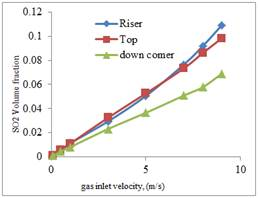Figure (3). SO2 volume fraction versus gas inlet velocity for different region.

In figure (4) superficial liquid velocities can be considered to flow up the riser virtually in plug flow. With increasing gas inlet velocities, the liquid velocities start to assume a parabolic profile.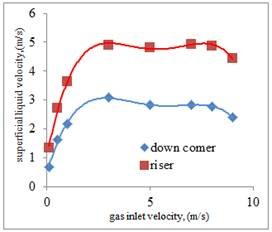Figure (4). superficial liquid velocity versus gas inlet velocity.

Within the central core of the riser, the gas holdup profiles are nearly uniform for the whole range of gas inlet velocities (ug) values. As shown in figure (5).Figure (5). SO2 volume fraction versus gas inlet velocity in riser.

Notably, the configurations of gas-liquid flow take place in the riser bubbly or bubbly turbulent flow. The transition gas holdup and gas inlet velocity identified using the drift flux plot, as shown in figure (6), where the change in the slope is the transition holdup (0.085) and from figure (5) the transient inlet gas velocity (5.79 m/s)Figure (6). Drift Flux versus Gas Holdup.

Interfacial area decreases when bubbles coalescence and increases when bubbles break up. In bubbly flow, it is considered that turbulent mixing of gas liquid interface is proportional to bubble diameter, dB and the turbulent velocity of gas phase is proportional to the turbulent velocity of liquid phase (Kataoka and Serizawa (1991a))As shown in figure (7)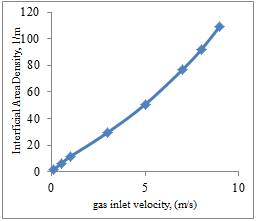Figure (7). Interfacial area density versus gas inlet velocity.

4. Conclusion

To conclude, there was an urgent need to address the emissions problems caused by Power plants and Oil industries and in order to find an innovative way and low-cost solutions. The researchers used the water as means for absorbing gases (sulfur dioxide) in the bubble column (airlift reactor). Recently, researchers have shown that the ability of water to dissolve sulfur dioxide and absorb enormous amounts of hazardous gases in ordinary conditions is considered an effective and low-cost solution.

Numerical calculations of gas inlet velocity shows declining in SO2 concentration in terms of degassing zone than draft tube region at high gas inlet velocity using drift flux method. The researchers observed the process region was bubbly and turbulent foamy. Interestingly, the researchers get perfect interfacial gas/liquid density with increasing of gas inlet velocity at uniform shape for the curve.

References

1. Bohn, M. (2000). "First 3D Simulation of Bubble Column Hydrodynamics Paves Way for Commercial Gas-to-Liquid Conversion in Slurry Reactor", Vice President, Engineering, 2000.
2. Buwa, V., and Ranade, V. (2003). "Bubbling Columns", National chemical laboratory, India, 2003.
3. Chisti. A., and Molina, D. (1998)." Areassessment of relationship between riser and downcomer gas holdups in airlift reactors ", Chem. Eng. Science, Vol. 53, No.24, pp.4151-4154, 1998.
4. Deen, N.G., Solberg, T., and Hjertager, B.H. (2000). "Numerical Simulation ofthe Gas-Liquid Flow in a Square Cross sectioned Bubble Column", http, 2000.
5. Freitas, J.A. and Teixeira, F. (1998)."Hydrodynamic studies in an airlift reactor with an enlarged degassing zone"; Bioprocess Engineering 18 (1998) 267±279 Ó Springer-Verlag
6. Krishna, R. and Baten, J. M. V. (2003). "Mass transfer of bubble columns", Catalysis Today. 79- 80: 67-75.
7. Lain, S.,Broder D., and Sommerfeld, M. (2000). "Numerical Modeling of the Hydrodynamics in a Bubble Column Using the Euler Lagrange Approach ", Universitat Halle-Wittenberg, D O6099 Halle (Saale). Germany.
8. Silva, R., Neto1, S., and Vilar, E. (2005). "A Computational Fluid Dynamics Study of Hydrogen Bubbles in an Electrochemical Reactor", Brazilian Archives of Biology and Technology Journal, Vol.48, Special n.: pp. 219-229, June 2005.
9. Van BatenJ.M. (2000). "CFD: a design and scale-up tool for multiphase reactors";PH.D Thesis; the institutional repository of the University of Amsterdam
10. ZHANG T., WANG T.,and WANG J. (2006). "Analysis And Measurement Of Mass Transfer In Airlift Loop Reactors"; Chinese J. Chem. Eng., 14(5) 604—610
11. Zhe, Cui. (2005). "Hydrodynamics In A Bubble Column At Elevated Pressures And Turbulence Energy Distribution In Bubbling Gas-Liquid And Gas-Liquid-Solid Flow Systems',; PH.D Thesis; The Ohio State University.

Article ToolsAbstractPDF(308K)